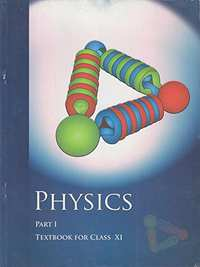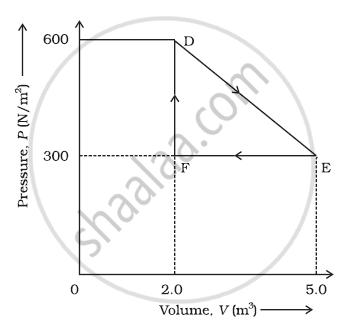# NCERT solutions for Class 11 Physics Textbook chapter 12 - Thermodynamics [Latest edition]

#### Chapters## Chapter 12: Thermodynamics

[Pages 316 - 317]

### NCERT solutions for Class 11 Physics Textbook Chapter 12 Thermodynamics [Pages 316 - 317]

Q 1 | Page 316

A geyser heats water flowing at the rate of 3.0 litres per minute from 27 °C to 77 °C. If the geyser operates on a gas burner, what is the rate of consumption of the fuel if its heat of combustion is 4.0 × 104 J/g?

Q 2 | Page 316

What amount of heat must be supplied to 2.0 x 10-2 kg of nitrogen (at room temperature) to raise its temperature by 45 °C at constant pressure? (Molecular mass of N2 = 28; R = 8.3 J mol-1 K-1.)

Q 3.1 | Page 316

Explain why Two bodies at different temperatures T1 and T2, if brought in thermal contact, do not necessarily settle to the mean temperature (T1 + T2)/2.

Q 3.2 | Page 316

The coolant in a chemical or a nuclear plant (i.e., the liquid used to prevent the different parts of a plant from getting too hot) should have high specific heat.

Q 3.3 | Page 316

Explain why Air pressure in a car tyre increases during driving.

Q 3.4 | Page 316

Explain why The climate of a harbour town is more temperate than that of a town in a desert at the same latitude.

Q 4 | Page 316

A cylinder with a movable piston contains 3 moles of hydrogen at standard temperature and pressure. The walls of the cylinder are made of a heat insulator, and the piston is insulated by having a pile of sand on it. By what factor does the pressure of the gas increase if the gas is compressed to half its original volume?

Q 5 | Page 316

In changing the state of a gas adiabatically from an equilibrium state to another equilibrium state B, an amount of work equal to 22.3 J is done on the system. If the gas is taken from state to via a process in which the net heat absorbed by the system is 9.35 cal, how much is the net work done by the system in the latter case? (Take 1 cal = 4.19 J)

Q 6 | Page 316

Two cylinders and of equal capacity are connected to each other via a stopcock. contains a gas at standard temperature and pressure. is completely evacuated. The entire system is thermally insulated. The stopcock is suddenly opened. Answer the following:

(a) What is the final pressure of the gas in and B?

(b) What is the change in internal energy of the gas?

(c) What is the change in the temperature of the gas?

(d) Do the intermediate states of the system (before settling to the final equilibrium state) lie on its P-V-T surface?

Q 7 | Page 317

A steam engine delivers 5.4×10J of work per minute and services 3.6 × 10J of heat per minute from its boiler. What is the efficiency of the engine? How much heat is wasted per minute?

Q 8 | Page 317

An electric heater supplies heat to a system at a rate of 100W. If the system performs work at a rate of 75 Joules per second. At what rate is the internal energy increasing?

Q 9 | Page 317

A thermodynamic system is taken from an original state to an intermediate state by the linear process shown in FigureIts volume is then reduced to the original value from E to F by an isobaric process. Calculate the total work done by the gas from D to E to F

Q 10 | Page 317

A refrigerator is to maintain eatables kept inside at 9°C. If the room temperature is 36° C, calculate the coefficient of performance.

In changing the state of a gas adiabatically from an equilibrium state to another equilibrium state B, an amount of work equal to 22.3 J is done on the system. If the gas is taken from state to via a process in which the net heat absorbed by the system is 9.35 cal, how much is the net work done by the system in the latter case? (Take 1 cal = 4.19 J)

## Chapter 12: Thermodynamics## NCERT solutions for Class 11 Physics Textbook chapter 12 - Thermodynamics

NCERT solutions for Class 11 Physics Textbook chapter 12 (Thermodynamics) include all questions with solution and detail explanation. This will clear students doubts about any question and improve application skills while preparing for board exams. The detailed, step-by-step solutions will help you understand the concepts better and clear your confusions, if any. Shaalaa.com has the CBSE Class 11 Physics Textbook solutions in a manner that help students grasp basic concepts better and faster.

Further, we at Shaalaa.com provide such solutions so that students can prepare for written exams. NCERT textbook solutions can be a core help for self-study and acts as a perfect self-help guidance for students.

Concepts covered in Class 11 Physics Textbook chapter 12 Thermodynamics are Isothermal Processes, Adiabatic Processes, Thermal Equilibrium, Zeroth Law of Thermodynamics, Heat, Internal Energy and Work, First Law of Thermodynamics, Specific Heat Capacity, Thermodynamic State Variables and Equation of State, Thermodynamic Processes, Heat Engines, Refrigerators and Heat Pumps, Second Law of Thermodynamics, Reversible and Irreversible Processes, Carnot Engine.

Using NCERT Class 11 solutions Thermodynamics exercise by students are an easy way to prepare for the exams, as they involve solutions arranged chapter-wise also page wise. The questions involved in NCERT Solutions are important questions that can be asked in the final exam. Maximum students of CBSE Class 11 prefer NCERT Textbook Solutions to score more in exam.

Get the free view of chapter 12 Thermodynamics Class 11 extra questions for Class 11 Physics Textbook and can use Shaalaa.com to keep it handy for your exam preparation